# Circular dendrogram in R with circlize and dendexten

Tal Galili

## Circular fan (polar) dendrogram plot

The `circlize_dendrogram` function can be used to create a circular dendrogram, also known as circular, radial or fan tree plot. You need to pass an object of class dendrogram. Note that `labels_track_height` and `dend_track_height` arguments are used to fit the labels properly and that both `circlize` and `dendextend` need to be installed to run the function.

``````# install.packages("dendextend")
# install.packages("circlize")
library(dendextend)
library(circlize)

# Distance matrix
d <- dist(USArrests)

# Hierarchical clustering dendrogram
hc <- as.dendrogram(hclust(d))

# Circular dendrogram
circlize_dendrogram(hc,
labels_track_height = NA,
dend_track_height = 0.5)``````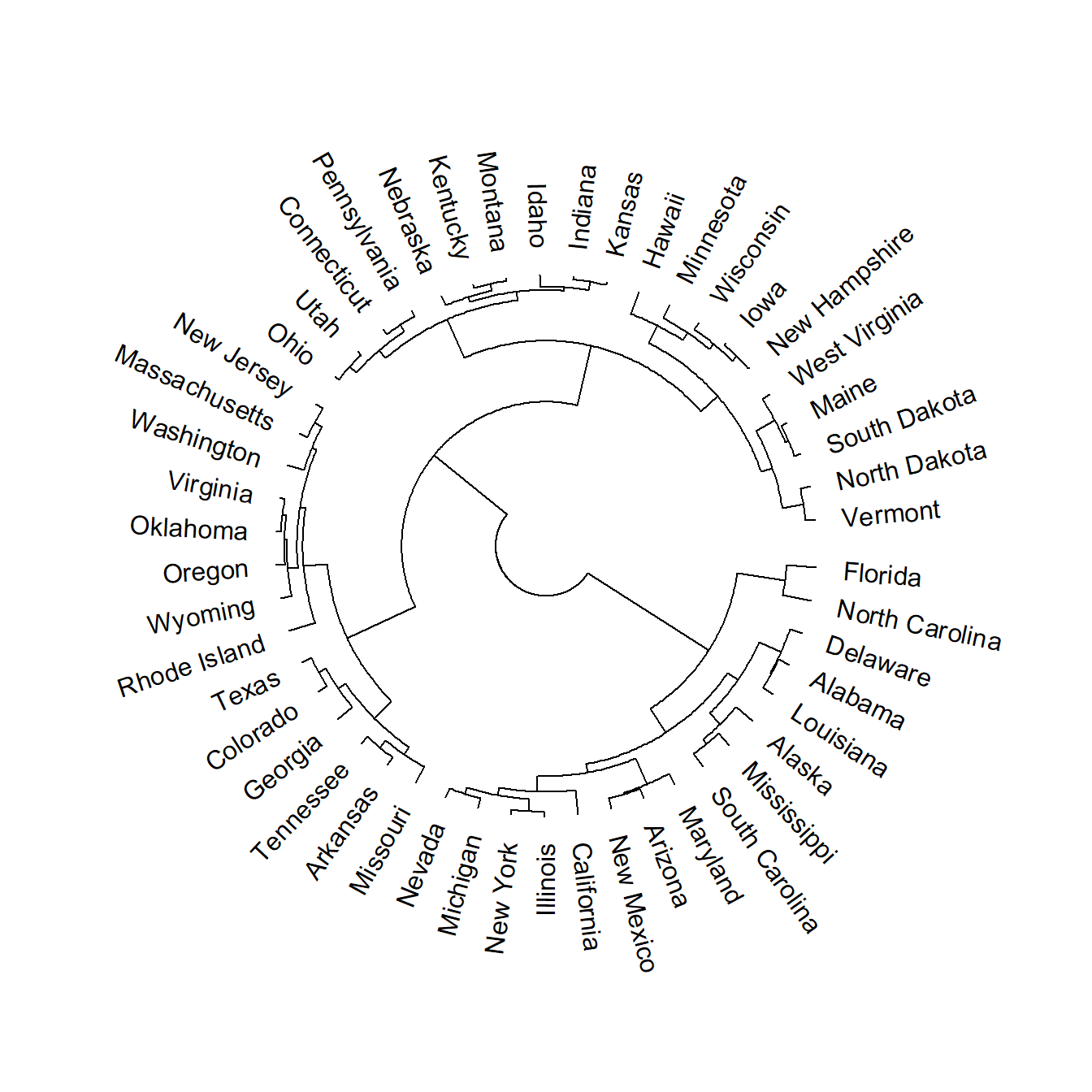The default dendrogram faces outside, but you can change this setting `facing = "inside"`.

``````# install.packages("dendextend")
# install.packages("circlize")
library(dendextend)
library(circlize)

# Distance matrix
d <- dist(USArrests)

# Hierarchical clustering dendrogram
hc <- as.dendrogram(hclust(d))

# Circular dendrogram facing inside
circlize_dendrogram(hc,
labels_track_height = NA,
dend_track_height = 0.5,
facing = "inside")``````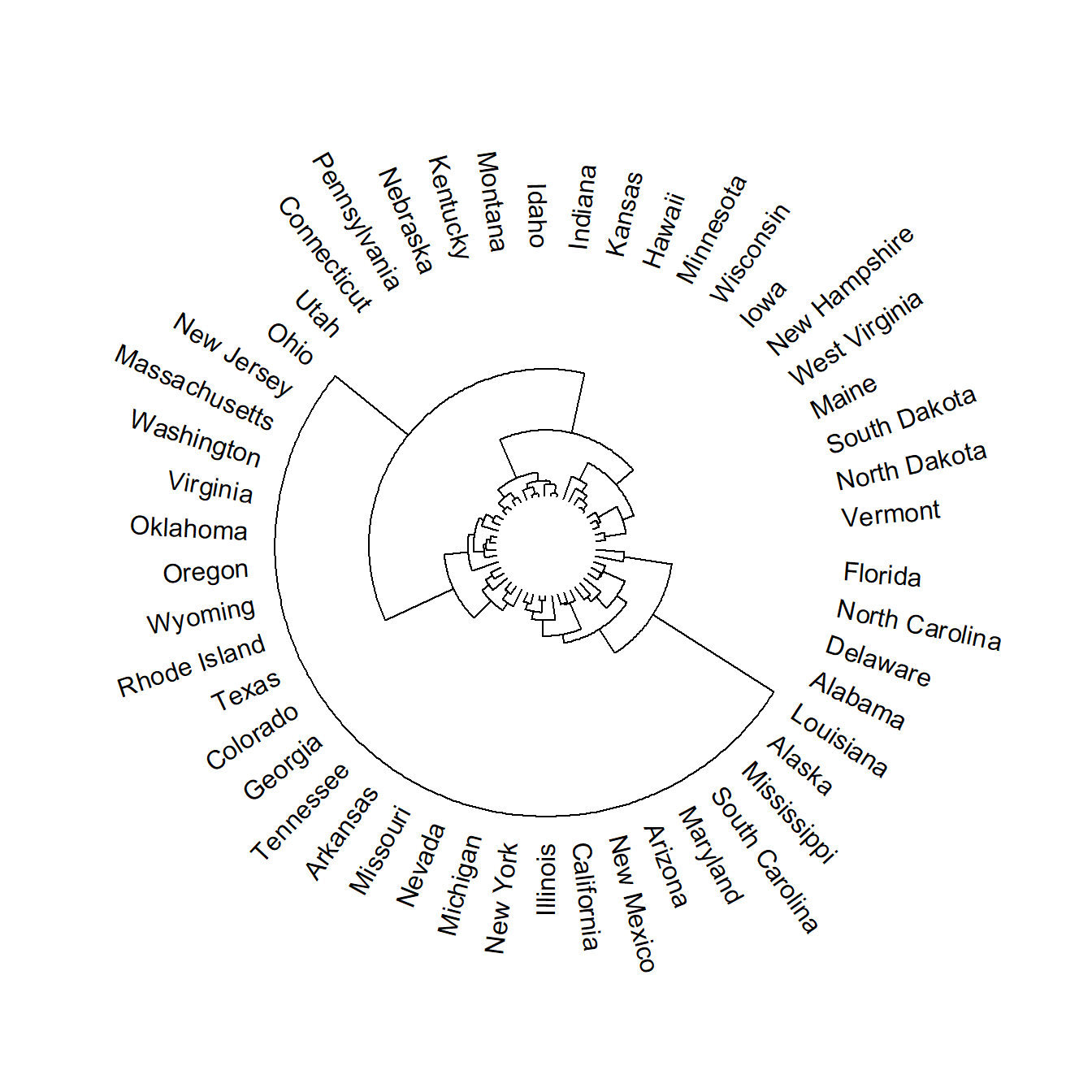The labels of the dendrogram can be removed if you only want to display the branches setting `labels = FALSE`. In this scenario is recommended to increase the `dend_track_height` argument value.

``````# install.packages("dendextend")
# install.packages("circlize")
library(dendextend)
library(circlize)

# Distance matrix
d <- dist(USArrests)

# Hierarchical clustering dendrogram
hc <- as.dendrogram(hclust(d))

# Circular dendrogram without labels
circlize_dendrogram(hc,
dend_track_height = 0.8,
labels = FALSE)``````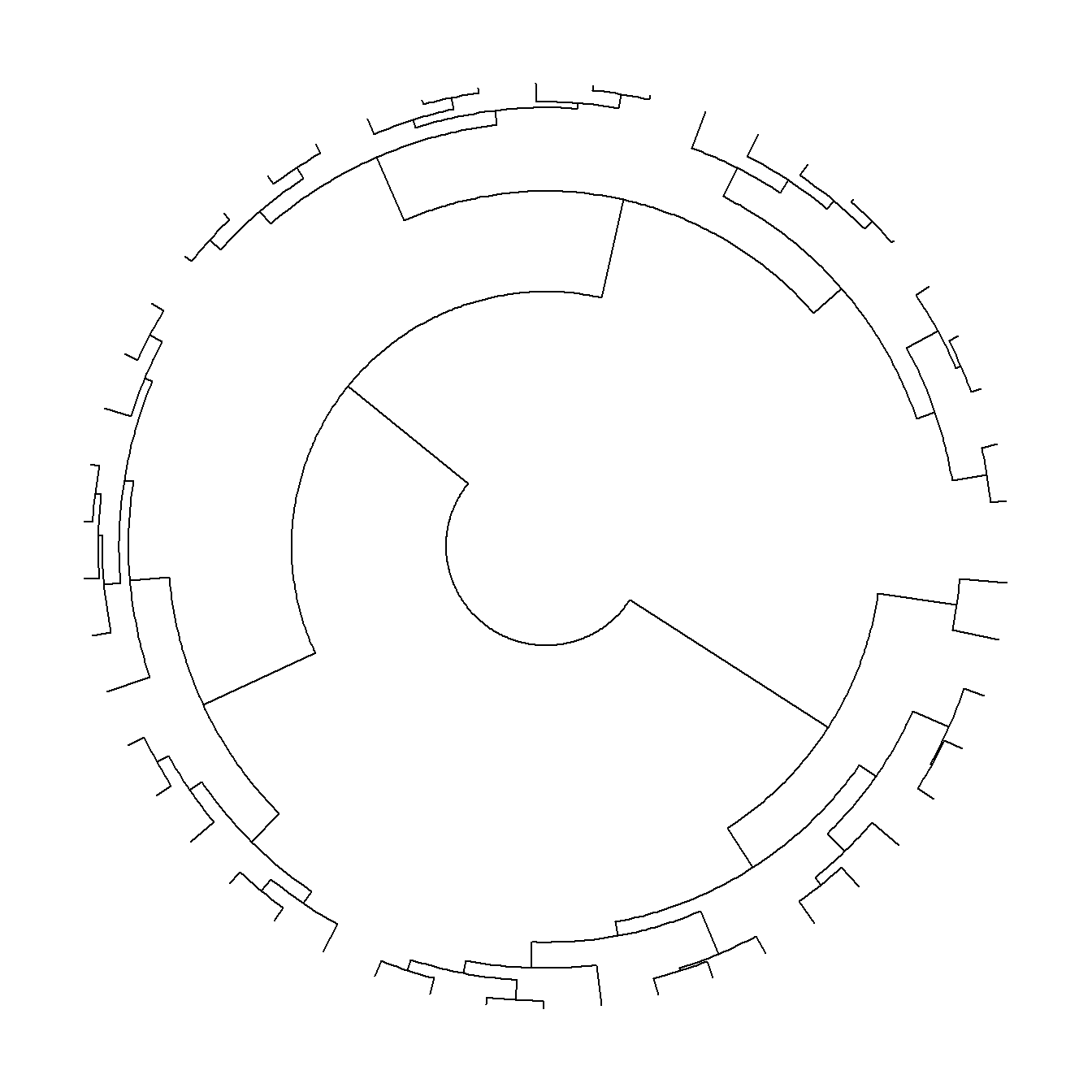## Further customization

The styling of the polar tree plot can be customized transforming the dendrogram object. If you want to colorize the branches you can use the `color_branches` function, based on a number of clusters (`k`) or a height (`h`). Note that the function contains an argument named `col` that allows customizing the colors.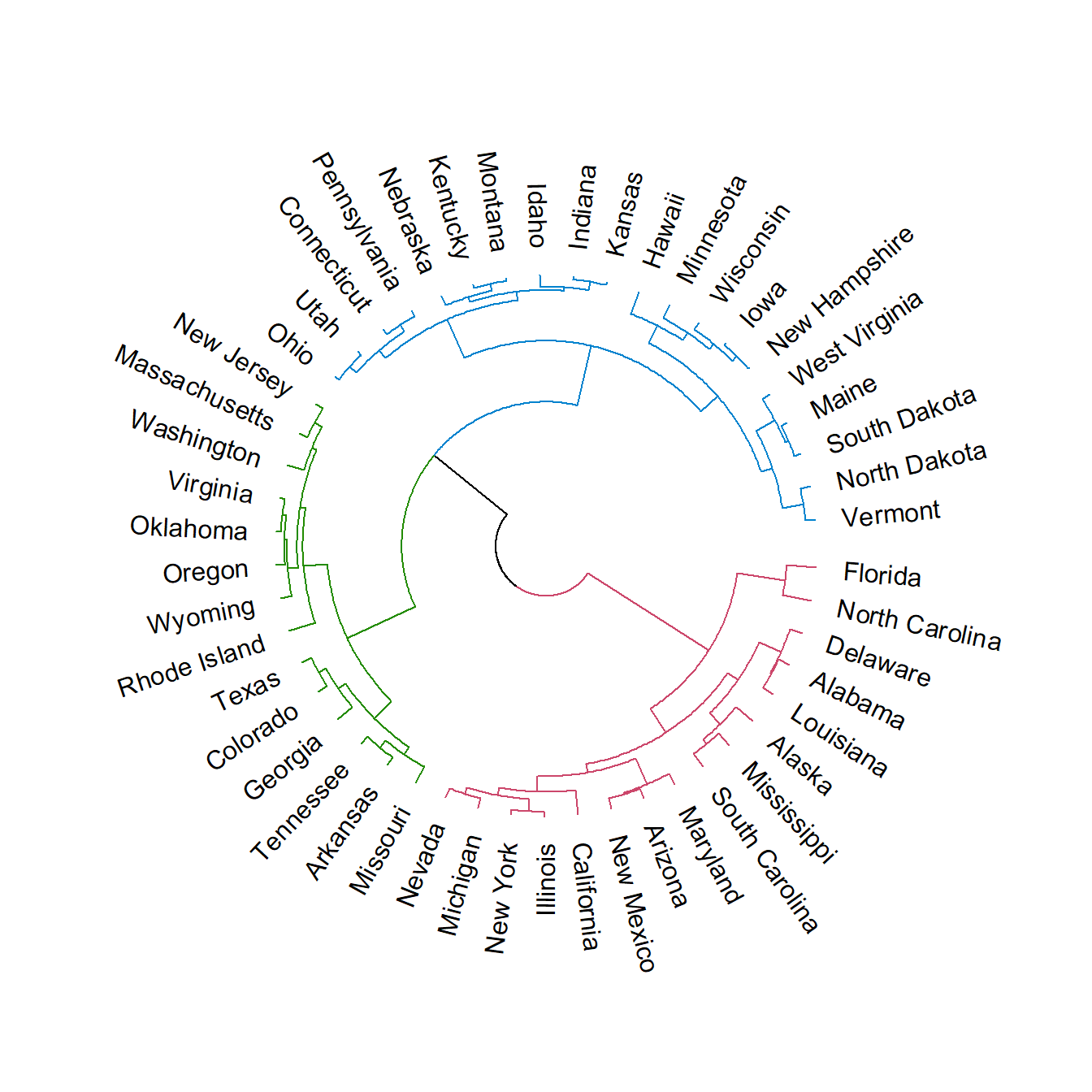``````# install.packages("dendextend")
# install.packages("circlize")
library(dendextend)
library(circlize)

# Distance matrix
d <- dist(USArrests)

# Hierarchical clustering dendrogram
hc <- as.dendrogram(hclust(d))

# Colors
hc <- hc %>%
color_branches(k = 3)
# hc <- color_branches(hc, k = 4)

circlize_dendrogram(hc,
labels_track_height = NA,
dend_track_height = 0.5)  ``````

The labels can also be customized the same way as the branches. For that purpose use the `color_labels` function and specify the number of clusters or the height.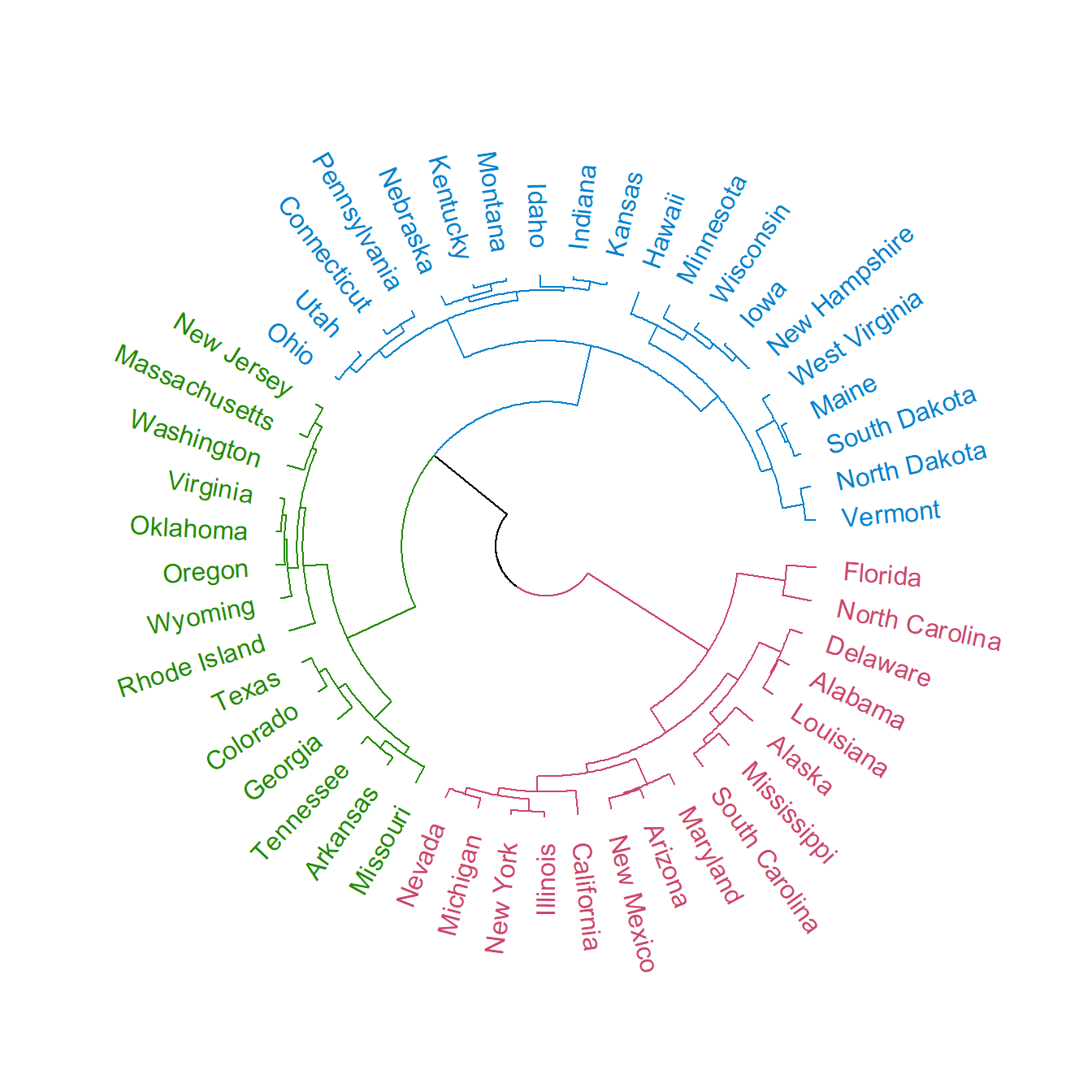``````# install.packages("dendextend")
# install.packages("circlize")
library(dendextend)
library(circlize)

# Distance matrix
d <- dist(USArrests)

# Hierarchical clustering dendrogram
hc <- as.dendrogram(hclust(d))

# Colors
hc <- hc %>%
color_branches(k = 3) %>%
color_labels(k = 3)

# Fan tree plot with colored labels
circlize_dendrogram(hc,
labels_track_height = NA,
dend_track_height = 0.5)  ``````

Finally, it is worth to mention that the style and width of the lines can be changed as in the example below.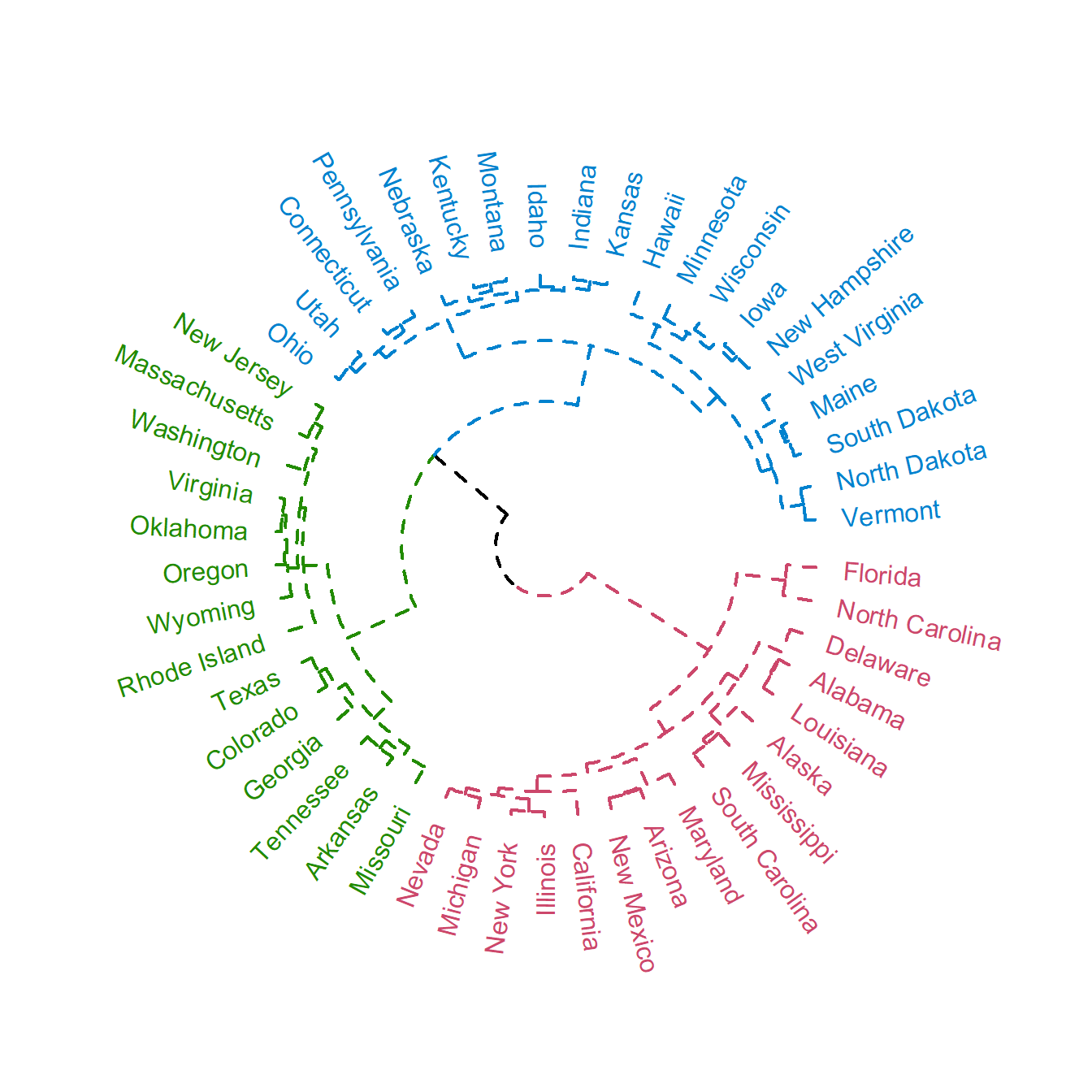``````# install.packages("dendextend")
# install.packages("circlize")
library(dendextend)
library(circlize)

# Distance matrix
d <- dist(USArrests)

# Hierarchical clustering dendrogram
hc <- as.dendrogram(hclust(d))

# Colors and line customization
hc <- hc %>%
color_branches(k = 3) %>%
set("branches_lwd", 2) %>%  # Line width
set("branches_lty", 2) %>%  # Line type
color_labels(k = 3)

# Line styling of the dendrogram
circlize_dendrogram(hc,
labels_track_height = NA,
dend_track_height = 0.5)  ``````